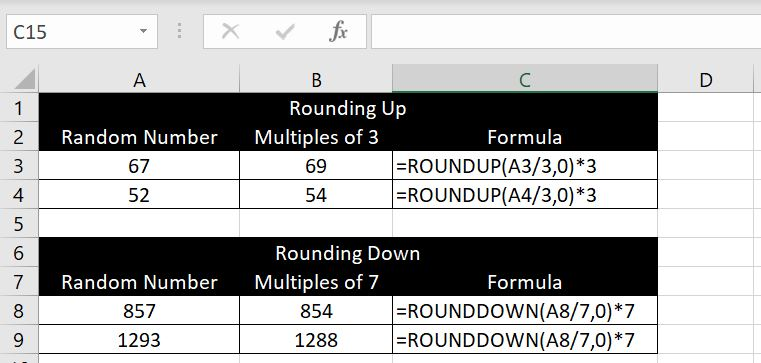## Rounding Numbers and Decimals in Excel

If you are interested in rounding numbers and decimals in Excel, you’ve found the right article. In this article, we will go through how to round decimal numbers up and down, specifying how many decimal places we want and also rounding numbers to the nearest multiples.

## Rounding Decimals – ROUND, ROUNDUP and ROUNDDOWN functions

There is a few Excel built-in functions that can be used to round up/down decimals and specify how many decimal places we want. Instead of going through them separately, we will explore them together in this section so we can see the differences and know when to use each one. There are three functions:

• ROUND, ROUNDUP, ROUNDDOWN

With each function, there are two variables that needs to be entered: 1) the number that needs rounding up/down and 2) the number of decimal places to be rounded to. Here’s the difference between the three:

1. ROUND: this function runs decimals up and down in accordance with the standard mathematical rules. For numbers 1 to 4, Excel would round the decimal down. For numbers 5 to 9, Excel would round the decimal up.
2. ROUNDUP: this function is different to above because it will always round a decimal up. If we use ROUNDUP with 1.51 and round to one decimal place, it will return with 1.6.
3. ROUNDDOWN: this function is the exact opposite of ROUNDUP. It will always round a decimal down. For example if we use ROUNDDOWN with 7.99 and round to one decimal place, it will return with 7.9.

Which one you use will depend on the situation. An example of where ROUNDUP could be useful would be parking fees in a carpark. We often pay for parking by the hour and as soon as we are one minute past the hour, we will need to pay for an extra full hour. For example, if you park in a carpark for 3 hours and one minute, you don’t pay for 3 hours and one minute, you pay for 4 hours. It rounds up.

Let’s have a look at a few examples here. The first picture below, we are using ROUND, ROUNDUP and ROUNDDOWN with the decimal number on the far left and for each function, we are rounding to two decimal places: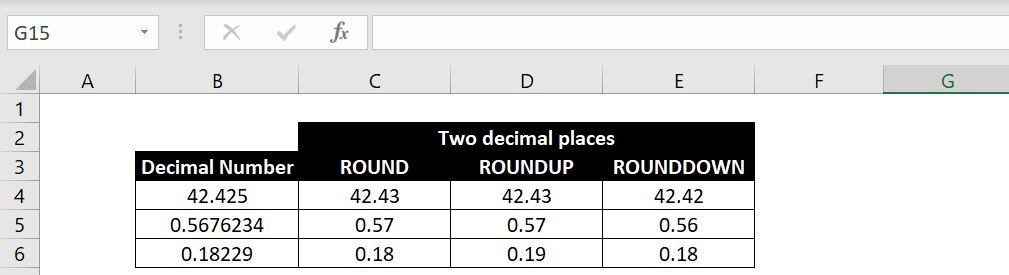Hopefully laying the functions and results side by side, you will be able to clearly see the differences. ROUND function should operate mathematically as we would all expect. With ROUNDUP, regardless what the 3rd decimal place is, the second decimal place will always go up by one. With ROUNDDOWN, regardless what the 3rd decimal place is, the second decimal place will always remain unchanged.

Here’s another example. In this case, we want 0 decimal place: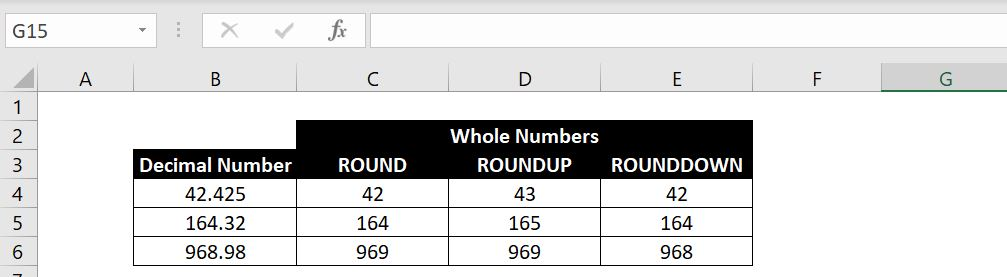The logic is the same. With ROUNDUP, regardless what the first decimal place is, it will always round the whole number up by one. With ROUNDDOWN, regardless what the first decimal place is, the whole number will always remain unchanged.

## Rounding Numbers to a Particular Multiple

In this section we will go through how to round numbers to a particular multiple. The function is MROUND. There are two variables required: 1) the number that needs rounding up/down and 2) the multiple to which the number is to be rounded to. We could specify any number and multiple and MROUND would round the number to the nearest multiple – up or down depending on which is closer.

In the examples below, for each number on the left, we are rounding it to the nearest multiples of 5s. For 1946, it is closer to 1945 than it is to 1950 so Excel returned 1945: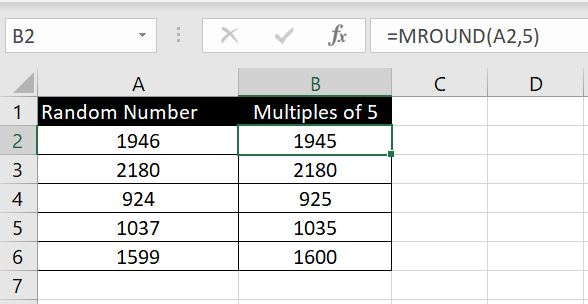And if the number is right in the middle between the two nearest multiples, it will be rounded up. In this example we are rounding each number to an even number. And in both cases, the number is rounded up to the next even number: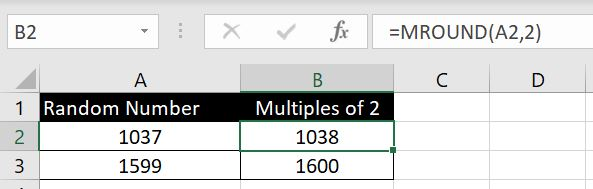### Choosing to Round Up or Down to a Particular Multiple

In the section and examples above, we can’t choose whether to round the number up or down. Excel does so for us depending which multiple is closer or in the situation where the number is in the middle, it rounds up to the higher multiple. What if we want to choose to always round up (or down) to the higher (or lower) multiple?

There is no built-in Excel function for this but the formula is not too complex:

• Rounding Up:
• = ROUNDUP(A2/n, 0) * n
• (n: the multiple)
• Rounding Down:
• = ROUNDDOWN(A2/n, 0) * n
• (n: the multiple)

The logic is simple. With rounding up, whatever the number is, we first divide it by the multiple. If it is divisible then no rounding is required. If it is not divisible, there will be a decimal. And from what we went through with ROUNDUP function above, if we set decimal place to 0, the number will always be rounded up to the next whole number. For example: 22.3 will become 23, and then we can multiply 23 by the multiple. This will always round the number up to the next multiple.

Same logic with ROUNDDOWN. Whatever decimal we get, the number will remain unchanged. For example: 122.43 becomes 122, and then we multiple 122 by the multiple. This will always round the number down to the lower multiple.

See example below: